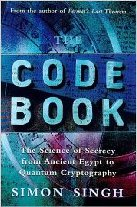## Introduction §

The straddling checkerboard is a substitution cipher, except that the substitutions are of variable length. It has formed a component of several impotant field ciphers, the most notable being the VIC cipher used by russian spies during the cold war.

When combined with other methods of encryption, as shown in the example, the straddling checkerboard can be quite strong.

If you are interested in breaking straddle checkerboard ciphers, check out over here.

## The Algorithm §

The key for a straddling checkerboard is a permutation of the alphabet e.g. 'fkmcpdyehbigqrosazlutjnwvx', along with 2 numbers e.g. 3 and 7. A straddling checkerboard is set up something like this (using the key information above):

```   0 1 2 3 4 5 6 7 8 9
f k m   c p d   y e
3: h b i g q r o s a z
7: l u t j n w v x
```

The first row is set up with the first eight key letters, leaving two blank spots. It has no row label. The second and third rows are labelled with whichever two digits didn't get a letter in the top row, and then filled out with the rest of the key letters. Since there are 30 slots in our grid, and we missed two letters in the first row, there will end up being two spare in the other rows. It doesn't matter where these spares go, so long as sender and receiver use the same system.

To encipher, a letter on the top row is simply replaced by the number labelling its column. Letters on the other rows are replaced by their row number, then column number:

```D E F E N  D T  H  E E A  S  T  W  A  L  L  O  F T  H  E C A  S  T  L  E
6 9 0 9 74 6 72 30 9 9 38 37 72 75 38 70 70 36 0 72 30 9 4 38 37 72 70 9
```

So, DEFEND THE EAST WALL OF THE CASTLE becomes 690975672309938377275387070360723094383772709. This may be sent directly, but usually is first input into a second cipher stage, such as a substitution or transposition step. As a simple example, we will add a secret key number (say, 83729) using non-carrying addition:

```  837298372983729837298372983729837298372983729
+ 690974672309938377275387070360723094383772709
= 427162944282657104463659953089550282655655428
```

Then use the same straddling checkerboard to turn it back into letters:

```427162944282657104463659953089550282655655428
CMU DMECCMYMDPU FCCDO PEEPH YEPPFMYMDPPDPPCMY
```

The final ciphertext is then CMUDMECCMYMDPUFCCDOPEEPHYEPPFMYMDPPDPPCMY. Note that it is a different length compared to the original plaintext. Deciphering is simply the reverse of this process. Although the size of groups can vary, deciphering is unambiguous because whenever the next element to be deciphered starts with a 3 or a 7, it is a pair; otherwise, it is a single letter.

## Javascript Example of the Straddling Checkerboard Cipher §

The following javascript example implements only the first step of the straddle checkerboard algorithm, i.e. the second stage (shown in the example) is not implemented.

Plaintext

key =
Spare positions:

Ciphertext

## Cryptanalysis §

The first step to breaking this cipher is to identify the blank positions in the key. This can often be done with frequency information i.e. if 2 digits are much more common than the rest, they are likely the first two blanks. The second two blanks can be put almost anywhere, as long as the cipher can be decrypted (i.e. there are no illegal sequences such as 38, 39, 78 or 79 for the example key above).

Once the blank positions are known, you can fill out the rest of the characters arbitrarily and decipher the ciphertext. This will leave you with a standard simple substitution cipher, which can be broken using standard techniques. This assumes you have the first two blanks in the right position.

This cipher is not terribly difficult to break using a computer, If you are interested in breaking straddle checkerboard ciphers, check out over here.

## ContentsThe Code Book: The Science of Secrecy from Ancient Egypt to Quantum Cryptography ASIN/ISBN: 978-1857028799 Simon Singh's 'The Code Book' is an excellent introduction to ciphers and codes Buy from Amazon.com